# 红外线透镜穿透率量测技术Measurement Technology of Transmittance of Infrared Optical System

DOI: 10.12677/OE.2020.101003, PDF, HTML, XML, 下载: 239  浏览: 1,331

Abstract: Infrared optical system is used in 8~12 μm range of infrared spectrum, which cannot be seen by human eyes, and the transmittance measurement method of visible optical system cannot be used, and the transmittance of infrared optical system was difficult to measure. At present, FTIR spec-trometer is used to measure transmittance of infrared optical system, which is very expensive and expensive to maintain. In this study, the transmittance of infrared optical system is measured by integrating the infrared sensing circuit and the smart infrared collimating beam. The measurement system consists of 2 mm diameter infrared collimating beam, thermopile sensor, OP amplifier, A/D IC and microprocessor. The thermopile converts the thermal radiation into analog electronic signal, and amplifies by OP amplifier, converted into digital by A/D IC, processed by 8051 microprocessor and displayed by LCD. The specifications of the lens to be tested are: focal length 19 mm, the f-number f/1.1 and the material of the lens was made by the black diamond. The test result of transmittance of the lens was 89%.

1. 前言

2. 基本原理  

$\text{TE:}\gamma \perp =\frac{\mathrm{cos}\theta -\sqrt{{n}^{2}-{\mathrm{sin}}^{2}\theta }}{\mathrm{cos}\theta +\sqrt{{n}^{2}-{\mathrm{sin}}^{2}\theta }}=-\frac{\mathrm{sin}\left({\theta }_{i}-{\theta }_{t}\right)}{\mathrm{sin}\left({\theta }_{i}+{\theta }_{t}\right)}$ (1)

$\text{TE:}\gamma \parallel =\frac{{n}^{2}\mathrm{cos}\theta -\sqrt{{n}^{2}-{\mathrm{sin}}^{2}\theta }}{{n}^{2}\mathrm{cos}\theta +\sqrt{{n}^{2}-{\mathrm{sin}}^{2}\theta }}=-\frac{\mathrm{tan}\left({\theta }_{i}-{\theta }_{t}\right)}{\mathrm{tan}\left({\theta }_{i}+{\theta }_{t}\right)}$ (2)

$\text{TE:}t\perp =\frac{2\mathrm{cos}\theta }{\mathrm{cos}\theta +\sqrt{{n}^{2}-{\mathrm{sin}}^{2}\theta }}=\frac{2\mathrm{sin}{\theta }_{t}\mathrm{cos}{\theta }_{i}}{\mathrm{sin}\left({\theta }_{i}+{\theta }_{t}\right)}$ (3)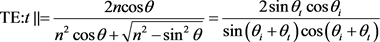(4)

${R}_{s}={r}_{s}{r}_{s}^{\varnothing },\text{\hspace{0.17em}}{R}_{p}={r}_{p}{r}_{p}^{\varnothing }$ (5)

${|{r}_{s}|}^{2}+{|{t}_{s}|}^{2}=1,\text{\hspace{0.17em}}{|{r}_{p}|}^{2}+{|{t}_{p}|}^{2}=1$ (6)

${R}_{s,normal}={R}_{p,normal}=\left[\frac{n-{n}^{\prime }}{n+{n}^{\prime }}\right]$ (7)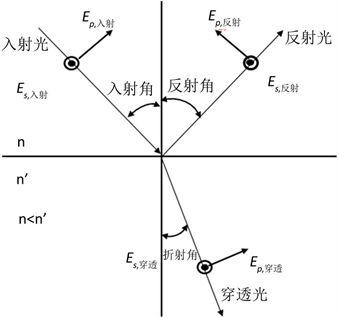Figure 1. Refraction and refraction at an interface between two media

3. 系统架构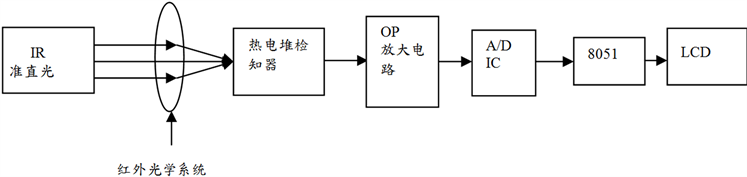Figure 2. Measurement system block diagram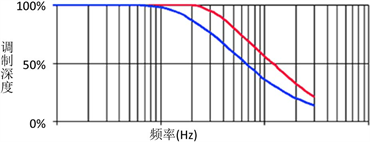(a)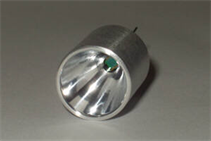(b)

Figure 3. (a) Thin film infrared source and parabolic mirror; (b) IR source modulation curveFigure 4. Thermopile sensorsTable 1. Optical electrical characteristics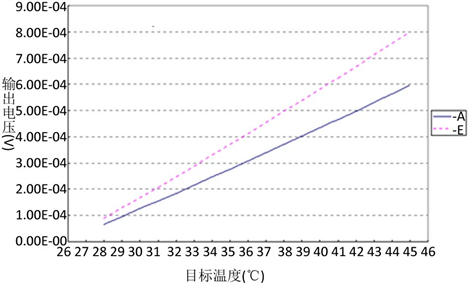Figure 5. Output and input curve

1) IR Source脉冲电路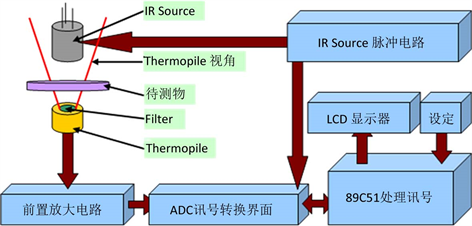Figure 6. System structureFigure 7. PWM modulations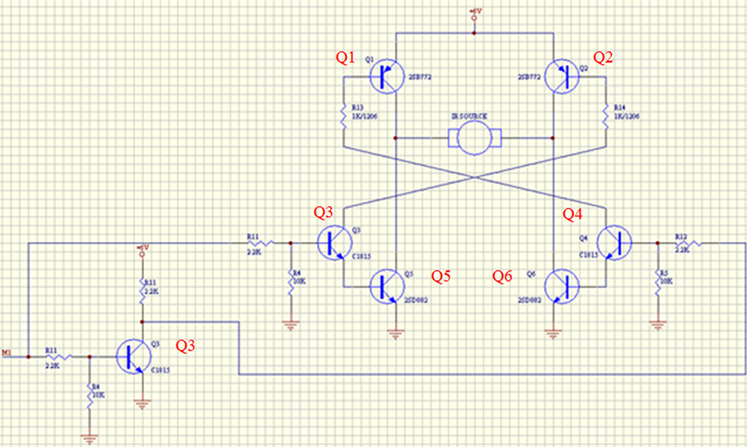Figure 8. IR source circuit

2) Thermopile的讯号放大

Thermopile的讯号输出大约只有数十μV~数mV左右，为了后续A/D数据撷取，必须进行高倍数的讯号放大。且因制程及材质的关系其内部阻抗也相当大，约在数十KΩ左右且变化很大，因此放大器的选择即是一项重要的工作。要选用适合的放大器，首先要考虑组件的特性。Thermopile的输出为一直流电压准位且压降微弱，只有数十μV~数mV左右，故选用的放大器带宽并不是我们在意的项目，必须特别注意的是VOS (Offset Voltage)这项参数。过大的VOS将会使电压输出失真，因此我们必须选用低VOS的运算放大器。另外VOS的温度飘移(Offset Drift)也应尽量选择低的参数值，以增加整个放大电路的稳定性。总和以上要点，我们选用了MAXIM的MAX4238，MAX4238的VOS在室温下的典型值只有0.1 μV，即使最大值也只有2 μV，且其VOS温度飘移为10 nV/℃。另外，MAX4238的增益带宽积(GBWP)也高达1 MHZ，在高倍数放大下也不至于失真，各规格特性均可完全符合我们的需求。

Thermopile的结构上约有数50~150 KΩ的内阻，且非定值，不同组件间的内阻差异也很大，为了排除Thermopile内部阻抗的影响，放大器电路设计上必须使用具有高输入阻抗的放大型式。非反向放大器即具有高输入阻抗的特性，此类型放大器输入阻抗一般可有数十MΩ~数百MΩ的等级。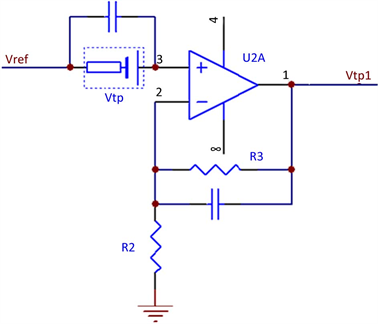Figure 9. Thermopile preamplifier circuit

$fc=\frac{1}{2\text{π}RC}=\frac{1}{2\text{π}×10\text{k}×1\text{μ}}=15.9\text{\hspace{0.17em}}\text{Hz}$ (8)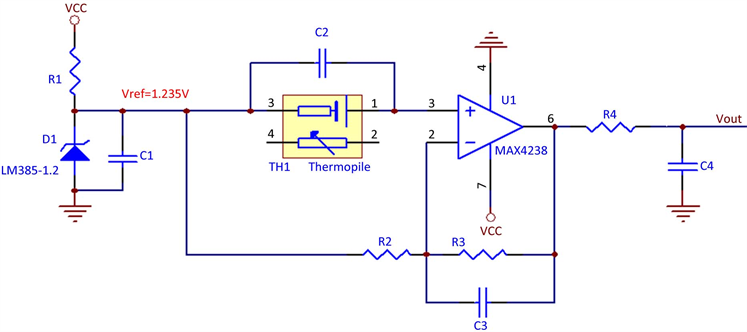Figure 10. Thermopile preamplifier circuit

MCP3302是一个SPI (Serial Peripheral Interface)串行接口的13bit SAR型模拟/数字转换器(A/D Converter)，可依需求使用4通道单端输入或2通道差动输入，电源电压使用5 V时，取样频率(sampling rate)可以达到100 KHZ，量测误差最大为±1 LSB INL (MCP3302-B)或±2 LSB INL (MCP3302-C)。

MCP3302使用上必须遵循其制定的SPI命令格式来对其进行转换与读取的动作。MCP3302的串行接口有三个入接脚，一个讯号输出接脚，其通讯格式里为一个启始位之后接着4个命令位，此4个位指定了MCP3302的动作模式，分别为1 bit的单端/差动选择位(Single/Diff)和3 bits的channel选择位(D2~D0)。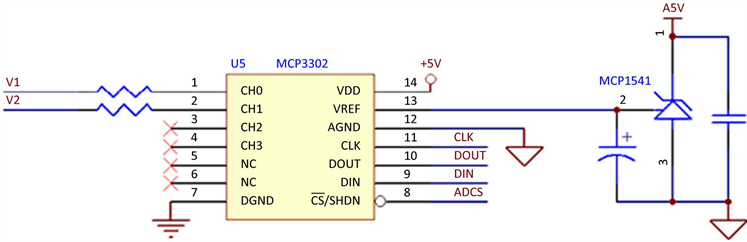Figure 11. ADC signal acquisition interface circuit

4) 单芯片89C51处理器

5) 红外线检测装置实体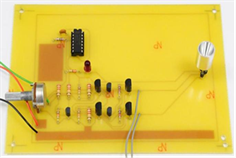(a)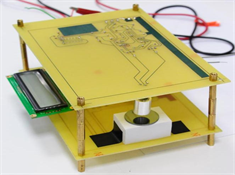(b)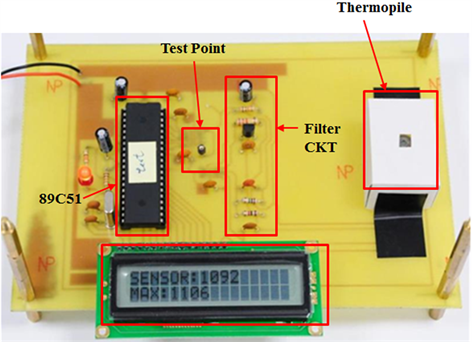(c)

Figure 12. (a) System hardware diagram; (b) IR Source pulse circuit; (c) Thermopile measurement circuit

4. 实验方法与结果

1) 测试物品：红外镜组焦距19 mm，其光圈f/1.1，光学材料为黑钻石硫属化物玻璃(Black Diamond™ chalcogenide glass)，其光学特性如表2所列。黑钻石硫属化物玻璃如AMTIR-1，IG6，BD2，GASIR-1及GE等其穿透率光谱范围0.7~20.0 μm如图13所示 。图14所示为红外线镜组外观图。

2) 精密镜片夹具：要求垂直度与平行度。

3) 红外光学系统穿透率量测系统。

4) 实验方法

a. 确认红外光学系统穿透率量测系统在最佳运作状况。

b. Alignment红外准直光束与Thermopile在同一水平面及同一光轴，使Thermopile输出信号最大。

c. 将红外镜组置于红外光学系统穿透率量测系统装置中。

d. 调整红外镜组垂直于远红外准直光束，平移Thermopile，使Thermopile接受到穿透信号最大。确认Thermopile接受到最大信号之后，进行穿透率量测。

e. 改变红外光源强弱，量取不同穿透信号。

f. 进行红外镜组穿透率分析。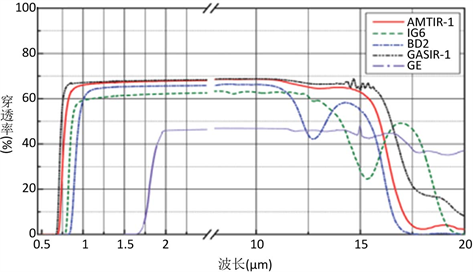Figure 13. Transmittance spectrum of the chalcogenide glassTable 2. Optical characteristics of infrared lens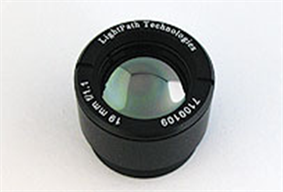Figure 14. Infrared lens

5) 红外镜组穿透率(T)的量测结果Table 3. Test data of transmittance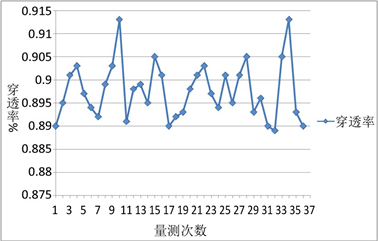Figure 15. Statistical curve of test data

5. 结论Table 4. Comparison of this method with FTIR spectrometer detection

  Hecht, E. (2016) Optics. 5th Edition, Addison-Wesley Longman, Inc., Reading.  Smith, W.J. (1992) Modern Lens Design. McGraw Hill, New York.  Index of /wp-content/uploads/Linear/IRSources. https://www.boselec.com/wp-content/uploads/Linear/IRSources  PerkinElmer Optoelectronics, Inc. (2003) “TPS 334—Thermopile Detector” Datasheet.  Wolfe, W.L., Kruse, P.W. and Bass, I.M. (1995) “Thermal detectors” in Handbook of Optics. McGraw-Hill, New York.  https://www.hamamatsu.com/resources/pdf/ssd/e07_handbook_Thermal_detectors, Chp. 7, pp. 3-7.  Nam, M., Washer, J. and Oh, J. (2015) Breaking the Mold: Overcoming Manufacturing Challenges of Chal-cogenide Glass Optics. https://www.photonics.com/Articles/Breaking_the_Mold_Overcoming_Manufacturing/a57309